Latest Banking jobs   »

# Reasoning Ability Quiz For FCI Phase I 2022- 23rd September

Q1. How many pairs of letters are there in the word ‘CHAMPIONS’, each of which have as many letters between then in the word as they have between then in the English alphabet?
(a) One
(b) Two
(c) Three
(d) Four
(e) None

Q2. The position of how many alphabets will remain unchanged if each of the alphabets in the word ‘OVERCOME’ is arranged in alphabetical order from left to right?
(a) Four
(b) Two
(c) Three
(d) One
(e) None

Q3. What will come in place of the question mark (?) to make the expressions I < H as well as K ≥ J definitely true? J = Y ≤ I ? K < H
(a) ≥
(b) <
(c) >
(d) ≤
(e) None of these

Directions (4-5): In each of the questions below some statements are given followed by some conclusions. You have to take the given statements to be true even if they seem to be at variance with commonly known facts. Read all the conclusions and then decide which of the given conclusions logically follows from the given statements, disregarding commonly known facts. Give answer

Q4. Statements: Some Pink are Black.
All Black are Yellow.
Some Blue are Black.
Conclusions: I. Some Yellow are Pink.
II. Some Blue being Pink is a possibility.
III. Some Yellow are not Blue.
(a) Both I and II follows
(b) Either I or II follows
(c) Only III follows
(d) None follows
(e) Only I follows

Q5. Statements: Only a few Mars are Venus.
All Mars are Star.
No Sun is Star.
Conclusions: I. All Venus is star is a possibility
II. Some Venus is Sun
III. No Mars is Sun
(a) Only I follow
(b) Only II follows
(c) Only III follows
(d) Both I and III follows
(e) None of these

Directions (6-10): Study the following information carefully and answer the given questions:
In a certain code language,
“Only in serial order” is written as “ve pu na to”,
“Order in the state” is written as “li ve su pu”,
“The logical idea only” is written as “su na ri jo”
“In idea or theory” is written as “zt jo bk pu”

Q6. Which of the following is the code of ‘or’?
(a) zt
(b) bk
(c) jo
(d) pu
(e) either ‘zt’ or ‘bk’

Q7. The code ‘li ri to ve’ may represent:
(a) serial order theory only
(b) only idea state order
(c) state logical serial order
(d) serial theory state the
(e) only the idea logical

Q8. Which of the following may represent ‘logical idea is order’?
(a) jo na ri ge
(b) ve na ri jo
(c) ri ve na zt
(d) bk to pu jo
(e) None of these

Q9. Which of the following is the code of ‘only’?
(a) su
(b) jo
(c) na
(d) ri
(e) Cannot be determined

Q10. Which of the following is code of ‘theory’?
(a) zt
(b) bk
(c) ve
(d) su
(e) Cannot be determined

Directions (11-15): Study the following information carefully and answer the questions given below:
In a certain code language
‘Study book room rent’ is coded as ‘dy bk rm rt’
‘Room rent is high’ is coded as ‘rm rt si gh’
‘Pay rent is more’ is coded as ‘yp rt si me’
‘More study work hard’ is coded as ‘me dy wk hd’

Q11. What is the code for ‘more room’?
(a) rm si
(b) me gh
(c) me rm
(d) bk rm
(e) None of these

Q12. What is the code for ‘work is hard’?
(a) hd gh si
(b) wk hd rt
(c) wk si hd
(d) si wk yp
(e) Cannot be determined

Q13. ‘dy’ is denoted as?
(a) book
(b) room
(c) rent
(d) study
(e) None of these

Q14. What is the code for ‘book’?
(a) dy
(b) bk
(c) rt
(d) rm
(e) None of these

Q15. ‘wk’ is the code for?
(a) work
(b) high
(c) hard
(d) Either (a) or (b)
(e) Cannot be determined

Solutions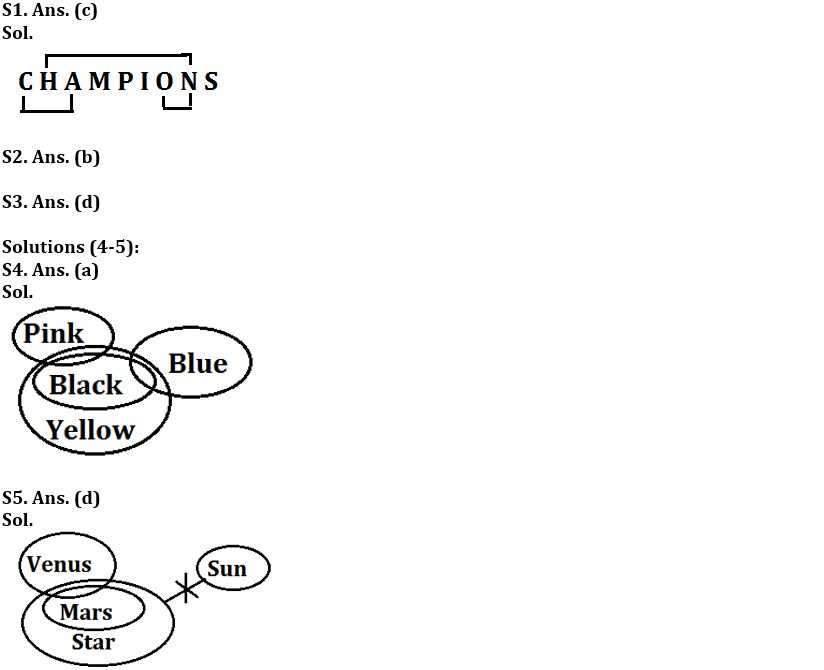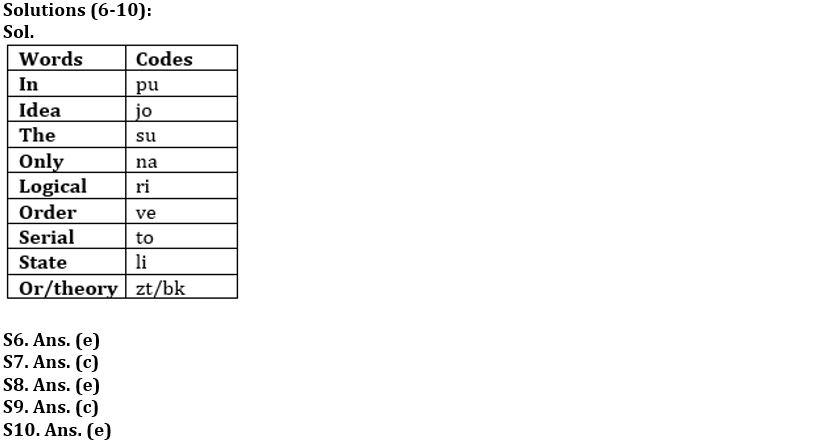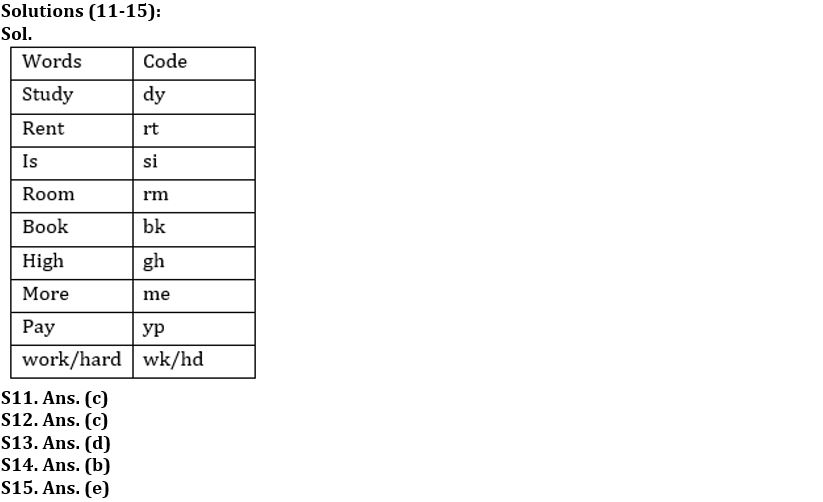#### Congratulations!Download Hindu Review of October 2021: Free PDFIncorrect details? Fill the form again here

Download Hindu Review of October 2021: Free PDF

Thank You, Your details have been submitted we will get back to you.
•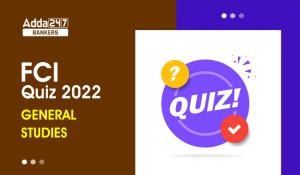General-Studies Quiz For FCI Phase I 202...
•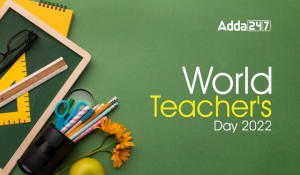World Teacher's Day 2022, Theme, History...
•Govt plans to set up 100 labs for 5G tec...
•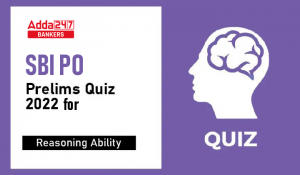Reasoning Ability Quiz For SBI PO Prelim...
•RBI Lists 16 NBFCs in Upper Layer Under ...
•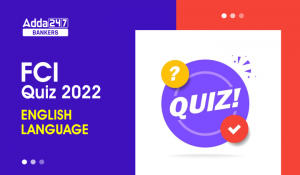English Quizzes For FCI Phase 1 2022- 3r...